### Home > A2C > Chapter Ch9 > Lesson 9.3.2 > Problem9-161

9-161.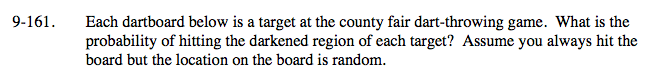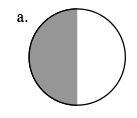Notice that half of the circle is shaded.

$\frac{1}{2}$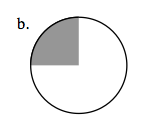See part (a).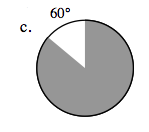Remember that 60° is one sixth of a full circle.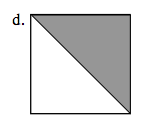See part (a).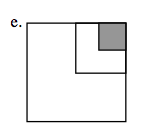Each of the smaller squares are a quarter of the next one larger than it.

$\frac{1}{16}$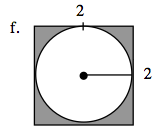Subtract the value of a circle with a radius of one from the 2 by 2 square.

4 − 1(π)

$1-\frac{\pi}{4}$